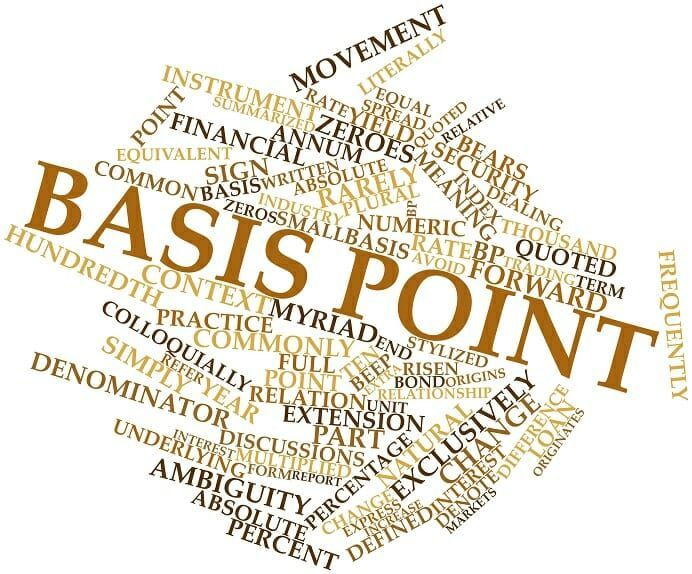# Basis Point (Beep)

Equivalent to one-hundredth of a percent or one ten-thousandth

## What is Basis Point (Beep)?

A Basis Point, often referred to as a Beep (using the notation bp), is a measurement of one-hundredth of a percent or one ten-thousandth and is a term commonly used in finance. Basis points are more commonly used in situations where differences of less than 1% are noteworthy. This is most often found to be true in fixed income markets.### Beeps in Relation to Interest Rates

Because the basis point is a convenient metric when discussing percentage changes that fall below 1%, it consistently comes up when talking about interest rates. While not always true, yearly changes in interest rates that don’t exceed a 1% difference are still typically meaningful for investors.

For example, consider that an interest rate of 3.50% climbs 0.10% for the year. It means that the interest rate increased by 10 basis points, leaving it at 3.60% at the end of the year. If, however, reports indicate that the same interest rate climbed 100 basis beeps for the year, it would mean a full percentage change, making the interest rate 4.50% by the end of the year.

### Common Uses of a Basis Point

The term basis point stems from trading the “basis” – the spread – between two interest rates. The basis (or spread) is generally small, leading it to be quoted in multiples of 10,000.

Specific loans and bonds are typically quoted using a relationship to an index or an underlying security or asset. In such cases, specifically, when the loan or bond is quoted in relation to a particular index, interest rates are quoted as a spread, either over or under the index. For example, consider that a bond with a yearly interest rate that is 0.25% higher than the London Interbank Offered Rate (LIBOR), which is among the most common benchmark interest rate indices. This means the basis or spread is indicated as 25 beeps over LIBOR.

When talking about forward forex markets, the basis point can be compared and contrasted with point in percentage (pip).

### Why is the Basis Point Important?

Aside from being able to calculate small percentage changes, as mentioned above, understanding and referencing basis points is also important because it helps remove ambiguity, especially when talking about interest rates and how they change. If an interest rate rises by 50 beeps, it rises by 0.50%.

The basis point is a very useful metric used to remove uncertainty when discussing rate changes and to identify and keep track of fractions of percentage changes.

Thank you for reading this guide to understanding what a beep is in finance. CFI is the official provider of the global Financial Modeling & Valuation Analyst certification program, designed to help anyone become a world-class financial analyst. See the following resources for more information:

• Effective Annual Interest Rate
• Inflation
• Bond Pricing
• Simple Interest

### Financial Analyst Training

Get world-class financial training with CFI’s online certified financial analyst training program!

Gain the confidence you need to move up the ladder in a high powered corporate finance career path.

Learn financial modeling and valuation in Excel the easy way, with step-by-step training.